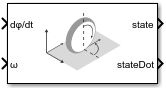# Unicycle Kinematic Model

Compute vehicle motion using unicycle kinematic model

• Library:
• Robotics System Toolbox / Mobile Robot Algorithms

•## Description

The Unicycle Kinematic Model block creates a unicycle vehicle model to simulate simplified car-like vehicle dynamics. This model approximates a vehicle as a unicycle with a given wheel radius, `Wheel radius`, that can spin in place according to a steering angular velocity, `ω`.

## Ports

### Input

expand all

Angular velocity of the wheel in radians per second.

#### Dependencies

To enable this port, set the ```Vehicle inputs``` parameter to ```Wheel Speed & Heading Angular Velocity```.

Vehicle speed, specified in meters per second.

#### Dependencies

To enable this port, set the ```Vehicle inputs``` parameter to ```Vehicle Speed & Heading Angular Velocity```.

Angular velocity of the vehicle, specified in radians per second. A positive value steers the vehicle left and negative values steer the vehicle right.

### Output

expand all

Current xy-position and orientation of the vehicle, specified as a [x y theta] vector in meters and radians.

The linear and angular velocities of the vehicle, specified as a [xDot yDot thetaDot] vector in meters per second and radians per second. The linear and angular velocities are calculated by taking the derivative of the `state` output.

## Parameters

expand all

Type of speed and directional inputs to control the vehicle. Options are:

• `Vehicle Speed & Heading Angular Velocity` — Vehicle speed in meters per second with a heading angular velocity in radians per second..

• `Wheel Speed & Heading Angular Velocity` — Wheel speed in radians per second with a heading angular velocity in radians per second.

The wheel radius of the vehicle, specified in meters.

The minimum and maximum wheel speeds, specified in radians per second.

The initial x-, `y`-position and orientation, theta, of the vehicle.

• `Interpreted execution` — Simulate model using the MATLAB® interpreter. For more information, see Simulation Modes (Simulink).

• `Code generation` — Simulate model using generated C code. The first time you run a simulation, Simulink® generates C code for the block. The C code is reused for subsequent simulations, as long as the model does not change.

Tunable: No

 Lynch, Kevin M., and Frank C. Park. Modern Robotics: Mechanics, Planning, and Control 1st ed. Cambridge, MA: Cambridge University Press, 2017.

##### Support평가판 신청